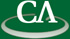## Finding Closed Form Solutions of Differential Equations

### Manuel Kauers, RISC Linz, Austria

Tuesday July 23rd, 2:30pm, IRMACS theatre, ASB 10900.

```Abstract: We consider ordinary linear differential equations with polynomial
coefficients. Each such equation has a finite dimensional vector space of
solutions, but usually none of these solutions can be expressed in closed
form. We discuss the problem of finding out for a given specific differential
equation whether one (or some, or all) of its solutions admit a closed form
representation. In the first part of the talk, we explain the classical algorithms
for finding polynomial and rational solutions. After that, we turn to hyperexponential
solutions. These are solutions that can be written in the form
\$\exp(u(x))v_1(x)^{e_1}\cdots v_k(x)^{e_k}\$ for certain rational functions
\$u,v_1,\dots,v_k\$ and constants \$e_1,\dots,e_k\$. There is a classical algorithm for
finding solutions of this form, but it is very expensive.
We present a more efficient algorithm, published a few weeks ago at ISSAC'13.

This is Joint work with F. Johansson and M. Mezzarobba.
```